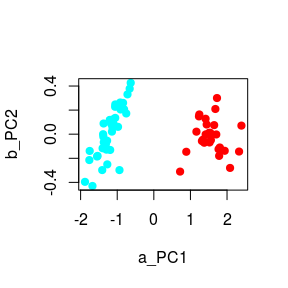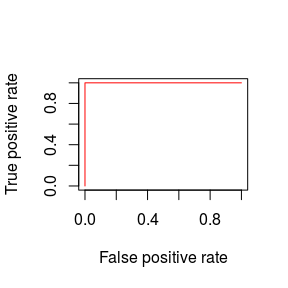# Advanced Topics for the exprso Package

## Foreword

Although this vignette contains a lot of exciting stuff, to get the most out of it, we recommend first reading the companion vignette, “An Introduction to the exprso Package”, which introduces many of the core features applied here.

## Tidy learning

All exprso modules support piping with the magrittr package. Piping is handled by two key functions, %>% and %T>%, that pass the result from a prior function call to the first argument of the next function call. However, the latter differs from the former in that it “branches out” and does not pass on its own result. Instead, %T>% pipes along the result from the previous function, making it useful for “side-chain” tasks like plotting. First, let us load some data.

library(exprso)
library(magrittr)
data(iris)
array <- exprso(iris[1:100, 1:4], iris[1:100, 5])
##  "Preparing data for binary classification."
##  "Converting binary labels to CONTROL / CASE."
##  "CONTROL: setosa (override with 'switch')"

Below, we use the %>% function to pre-process the data and split it into a training and test set. Since the data object forks at the level of the split method (yielding two ExprsArray objects from one), it makes sense to break the pipe cascade there.

splitSets <- array %>%
modTransform %>% modNormalize %>%
splitSample(percent.include = 67)

Next, we use the %>% function to pull the training set from the split method result (via the trainingSet function) and pipe it through a chain of feature selection and classifier construction methods. Similar to trainingSet, the testSet function (or, equivalently, the validationSet function) will extract the test set from the split method result.

pred <- trainingSet(splitSets) %>%
fsStats(how = "t.test") %>%
fsPrcomp(top = 2) %T>%
plot(c = 0) %>%
buildSVM %>%
predict(testSet(splitSets)) %T>%
calcStatsPiping can expedite ensemble classifier construction as well. Here, we use the %>% function in conjunction with plMonteCarlo to (a) split the training set across 10 bootstraps, (b) perform recursive feature elimination on each training subset, (c) construct an LDA classifier, and (d) deploy the classifier on an internal validation set. Then, we select the best three performing classifiers, regardless of the bootstrap origin, by passing the results through pipeUnboot and pipeFilter (see ?pipeUnboot and ?pipeFilter to learn more about how the “boot” column changes pipeFilter behavior). Last, we build a classifier ensemble and deploy it on the test set. For code clarity, we define the argument handler functions ctrlSplitSet, ctrlFeatureSelect, and ctrlGridSearch outside of the pipe cascade.

ss <- ctrlSplitSet(func = "splitSample", percent.include = 67, replace = TRUE)
fs <- ctrlFeatureSelect(func = "fsPathClassRFE", top = 0)
gs <- ctrlGridSearch(func = "plGrid", top = 0, how = "buildLDA")

pred <- trainingSet(splitSets) %>%
plMonteCarlo(B = 10, ctrlSS = ss, ctrlFS = fs, ctrlGS = gs) %>%
pipeUnboot %>%
pipeFilter(colBy = "valid.auc", top = 3) %>%
buildEnsemble %>%
predict(testSet(splitSets)) %T>%
calcStats

## Clustering before classifying

The exprso package also includes an experimental function, modCluster, that clusters subjects prior to building models. This function uses the how argument to toggle between one of seven clustering algorithms and returns an ExprsArray object with updated @annot slot that contains the results of clustering.

pred <- trainingSet(splitSets) %>%
modCluster(top = 0, how = "hclust", k = 4) %>%
modSubset(colBy = "cluster", include = 1)

Next, we show how to make a custom training set based on a cluster of cases and all controls. For this, we use modCluster in conjunction with the conjoin function. Note that using conjoin after feature selection will throw an error. Although we cluster cases here, this technique would work for any data annotation.

clusteredCases <- trainingSet(splitSets) %>%
modSubset(colBy = "defineCase", include = "Case") %>%
modCluster %>%
modSubset(colBy = "cluster", include = 1) %>%
conjoin(trainingSet(splitSets) %>%
modSubset(colBy = "defineCase", include = "Control"))

## Importing GSE files

The NCBI GEO hosts files in GSE or GDS format, the latter being a curated version the former. These GDS data files easily convert to an ExpressionSet (abbreviated eSet) object using the GDS2eSet function from the GEOquery package. However, not all GSE data files have a corresponding GDS data file available. Instead, we can use the GSE2eSet function to build an eSet object from any GSE data file. The arrayExprs function imports an eSet object into exprso.

data.gse <- GEOquery::getGEO("GSE5847", GSEMatrix = FALSE)
data.eset <- GSE2eSet(data.gse)
data.eset@phenoData@data

## Deep learning

Deep learning in exprso does not differ much from the other approaches to classification. However, supplying arguments can get cumbersome.

pred <- trainingSet(splitSets) %>%
buildDNN(top = 0,
activation = "TanhWithDropout", # or 'Tanh'
input_dropout_ratio = 0.2, # % of inputs dropout
hidden_dropout_ratios = c(0.5,0.5,0.5), # % for nodes dropout
balance_classes = TRUE,
hidden = c(50,50,50), # three layers of 50 nodes
epochs = 100) %>%
predict(testSet(splitSets)) %T>%
calcStats

One important difference with buildDNN is that you must manually clear the old classification models from RAM. Unlike with other models, the ExprsModel object does not actually store the deep neural net, but rather just holds a “link” to the actual classifier which is stored outside of R.

h2o::h2o.shutdown() # frees up RAM for more learning

When embedding buildDNN within a grid-search, we run into the difficulty that most buildDNN arguments require a numeric vector as input. These vector inputs typically correspond to a unique value for each layer of the deep neural net. We can provide plGrid a vector argument by wrapping it in a list. Take note that this approach of providing argument vectors in a list would also work for other arguments (e.g., character arguments to top).

pl <- trainingSet(splitSets) %>%
plGrid(array.valid = testSet(splitSets), top = 0,
how = "buildDNN", fold = NULL,
activation = "TanhWithDropout", # or 'Tanh'
input_dropout_ratio = 0.2, # % of inputs dropout
hidden_dropout_ratios = list(c(0.5,0.5,0.5)), # % for nodes dropout
balance_classes = TRUE,
hidden = list(c(50,50,50)), # three layers of 50 nodes
epochs = 100)

Below, we show a more elaborate deep learning grid-search. For details on these arguments, see ?h2o::h2o.deeplearning.

pl <- trainingSet(splitSets) %>%
plGrid(array.valid = testSet(splitSets), top = 0,
how = "buildDNN", fold = NULL,
activation = c("Rectifier",
"TanhWithDropout"), # or 'Tanh'
input_dropout_ratio = c(0.2,
0.5,
0.8), # % of inputs dropout
hidden_dropout_ratios = list(c(0.5,0.5,0.5),
c(0.2,0.2,0.2)), # % for nodes dropout
balance_classes = TRUE,
hidden = list(c(50,50,50),
c(100,100,100),
c(200,200,200)), # three layers of 50 nodes
epochs = c(100))

Keep in mind that deep learning is a very RAM hungry task. If you’re not careful, you’ll run out RAM and throw an error. Remember to call h2o::h2o.shutdown() whenever you finish!

## Perfect cross-validation

The “perfect” cross-validation pipeline would have two layers of cross-validation such that the outer-layer divides the data without feature selection while the inner-layer divides the data with feature selection. We can achieve this in exprso by embedding a plNested pipeline within another plNested pipeline.

If you use this approach, you should not need a test set as long as you calculate classification accuracy in a way that respects the independence of each fold. In other words, if you opt out of a test set, you must never let a validation set accuracy guide the selection of which training sets to use when calculating the final classification accuracy.

For illustrative purposes, we perform “perfect” cross-validation using support vector machines built across a single set of parameters. Extending this pipeline to a larger parameter grid-search will require a cautious analysis of the results.

fs.inner <- ctrlFeatureSelect(func = "fsStats", top = 0, how = "t.test")
gs.inner <- ctrlGridSearch(func = "plGrid", top = 3,
how = "buildSVM", fold = NULL)

fs.outer <- ctrlFeatureSelect(func = "fsNULL", top = 0)
gs.outer <- ctrlGridSearch(func = "plNested", fold = 2,
ctrlFS = fs.inner, ctrlGS = gs.inner)

pl <- array %>%
modTransform %>% modNormalize %>%
plNested(fold = 2, ctrlFS = fs.outer, ctrlGS = gs.outer)

## Cross-validation variants

Typically, we summarize rounds of cross-validation using accuracy. However, we could conceive of situations where we might want to emphasize sensitivity over specificity (or vice versa). Below, we show how we can use plNested in lieu of plCV to select plMonteCarlo bootstraps based on sensitivity.

In this example, each iteration of plMonteCarlo will split the dataset, then call plNested on the training subset. Next, plNested will manage $$v$$-fold cross-validation, splitting the data into $$v$$ equal folds. Finally, each fold will undergo a grid-search according to plGrid. Since we have chosen to use plNested in lieu of plCV, we disable plCV by setting the plGrid argument fold = NULL. Note that, as above, we only perform feature selection within the inner-layer. This ensures that the outer-layer serves as a truly independent validation set.

fs.inner <- ctrlFeatureSelect(func = "fsStats", top = 0, how = "t.test")
gs.inner <- ctrlGridSearch(func = "plGrid", top = c(2, 3, 4),
how = "buildSVM", fold = NULL)

ss.outer <- ctrlSplitSet(func = "splitStratify", percent.include = 67)
fs.outer <- ctrlFeatureSelect(func = "fsNULL", top = 0)
gs.outer <- ctrlGridSearch(func = "plNested", fold = 10,
ctrlFS = fs.inner, ctrlGS = gs.inner)

pl <- array %>%
modTransform %>% modNormalize %>%
plMonteCarlo(B = 5, ctrlSS = ss.outer, ctrlFS = fs.outer, ctrlGS = gs.outer)

The resultant object now contains the necessary information to rank plMonteCarlo bootstraps based on $$v$$-fold cross-validation sensitivity or specificity. Alternatively, we could aggregate the results by selecting the best fold from each bootstrap using pipeFilter, emphasizing sensitivity over specificity with colBy.

top <-
pipeFilter(pl, colBy = c("valid.sens", "valid.sens", "valid.spec"), top = 1)

## Multi-class classification

The exprso package also contains a growing number of methods made specifically for dealing with multi-class data. For example, all of the build methods available for binary classification also work for multi-class classification. In addition, exprso also contains some feature selection methods that work for binary and multi-class data alike.

Below, we use mock multi-class data to illustrate a simple multi-class classification pipeline.

splitSets <- data(arrayMulti) %>% get %>%
splitStratify(percent.include = 67, colBy = "sex")

trainingSet(splitSets) %>%
fsANOVA %>%
buildNB %>% # any build method can become multi with 1-vs-all
predict(testSet(splitSets)) %T>%
calcStats

All the pipelines developed for binary classification work equally well for multi-class classification. However, not all feature selection methods work for multi-class data. As long as you choose a valid multi-class feature selection method, plGrid, plMonteCarlo, and plNested will work without fail.

fs <- ctrlFeatureSelect(func = "fsANOVA", top = 0)
gs <- ctrlGridSearch(func = "plGrid", top = 0, how = "buildRF", fold = 2)

pl <- trainingSet(splitSets) %>%
plNested(fold = 2, ctrlFS = fs, ctrlGS = gs) %T>%
calcNested(colBy = "valid.acc")

Note that exprso also supports multi-class classifier ensembles.

pl %>% buildEnsemble %>%
predict(testSet(splitSets)) %>%
calcStats

A special plGrid variant, called plGridMulti, is also available for multi-class data. This variant uses 1-vs-all feature selection instead of multi-class feature selection. In this implementation, 1-vs-all feature selection occurs just prior to 1-vs-all classifier construction. As such, each individual ExprsMachine within the ExprsModule will have its own unique feature selection history to pass on to the test set during classifier deployment. For plGridMulti, the 1-vs-all feature selection is managed just like the other pl functions, using the ctrlFeatureSelect argument handler.

fs <- ctrlFeatureSelect(func = "fsStats", top = 0, how = "t.test")

pl <- plGridMulti(trainingSet(splitSets), testSet(splitSets),
ctrlFS = fs, top = c(2, 3),
how = "buildSVM", kernel = c("linear", "radial"),
gamma = c(.1, .2))

However, plGridMulti does not have built-in plCV support. Instead, use plNested.

fs.inner <- ctrlFeatureSelect(func = "fsStats", top = 0, how = "t.test")
fs.outer <- ctrlFeatureSelect(func = "fsNULL", top = 0)
gs.outer <-
ctrlGridSearch(func = "plGridMulti", ctrlFS = fs.inner, top = c(2, 3),
how = "buildSVM", kernel = c("linear", "radial"), gamma = c(.1, .2))

pl <- plNested(trainingSet(splitSets), fold = 2,
ctrlFS = fs.outer, ctrlGS = gs.outer)